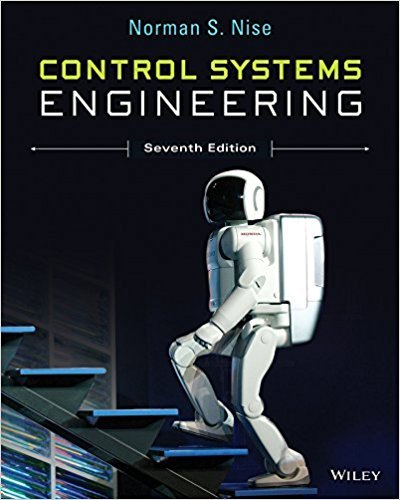×
Get Full Access to Control Systems Engineering - 7 Edition - Chapter 2 - Problem 11
Get Full Access to Control Systems Engineering - 7 Edition - Chapter 2 - Problem 11

×

# Write the differential equation that is mathematicallyISBN: 9781118170519 162

## Solution for problem 11 Chapter 2

Control Systems Engineering | 7th Edition

• Textbook Solutions
• 2901 Step-by-step solutions solved by professors and subject experts
• Get 24/7 help from StudySoup virtual teaching assistantsControl Systems Engineering | 7th Edition

4 5 1 384 Reviews
14
0
Problem 11

Write the differential equation that is mathematically equivalent to the block diagram shown in Figure P2.2. Assume that rt 3t 3. [Section: 2.3] s4 + 3s3 + 2s2 + s + 1 s5 + 4s4 + 3s3 + 2s2 + 3s + 2 R(s) C(s) FIGURE P2.2

Step-by-Step Solution:
Step 1 of 3

Step 2 of 3

Step 3 of 3

##### ISBN: 9781118170519

Unlock Textbook Solution